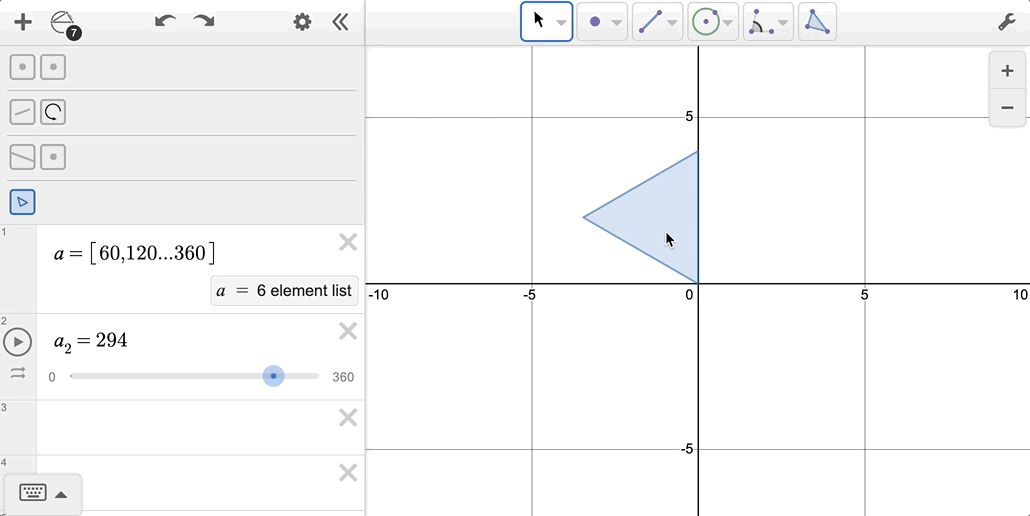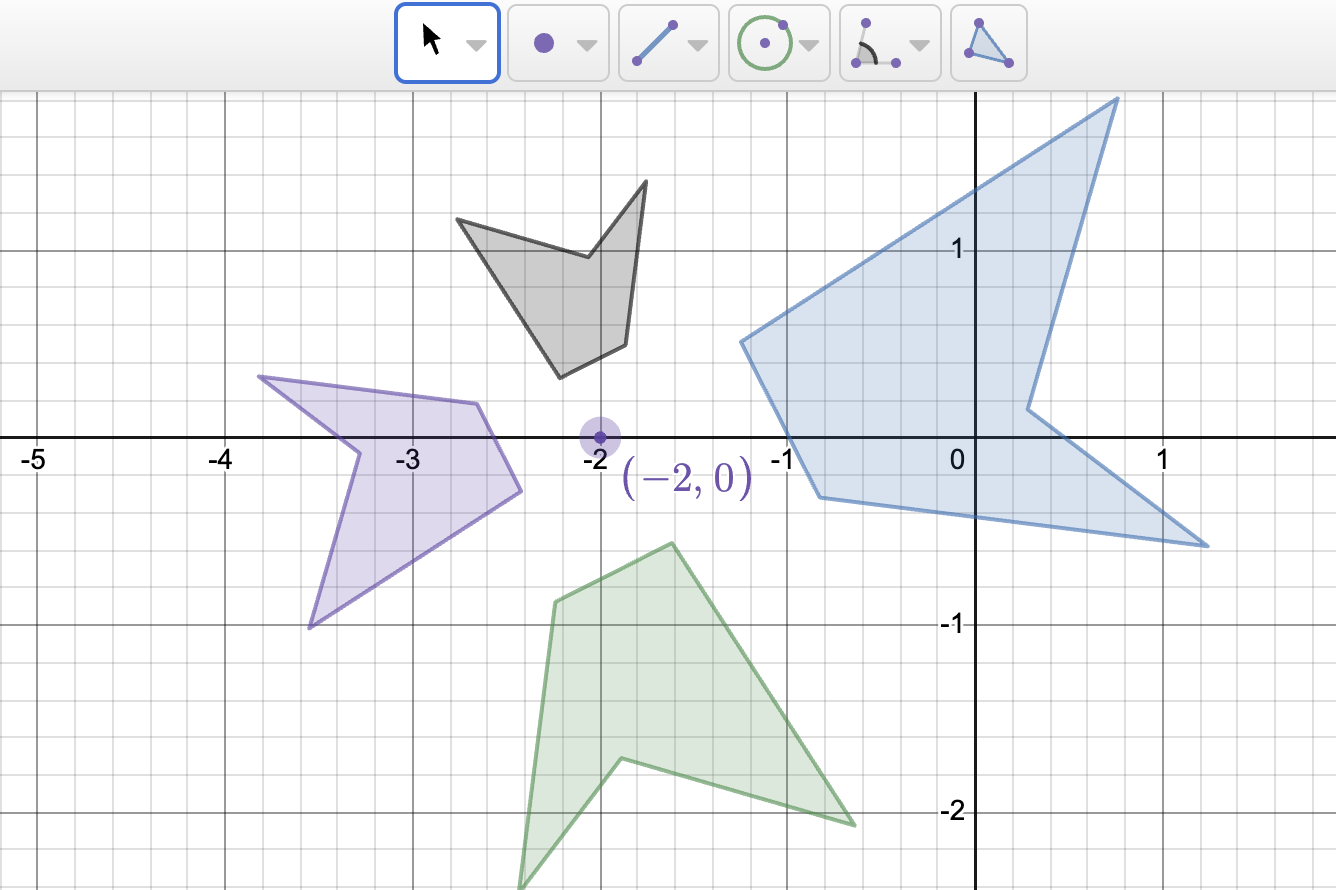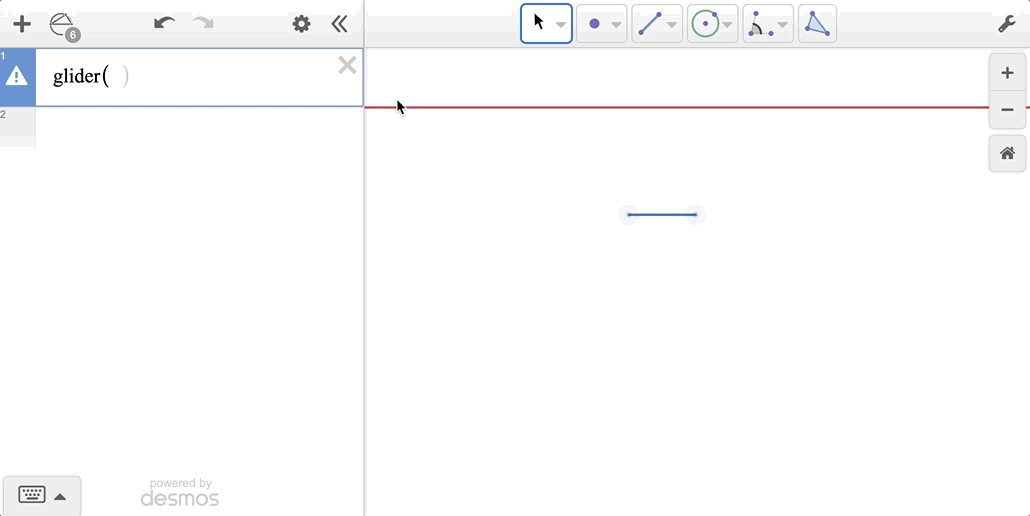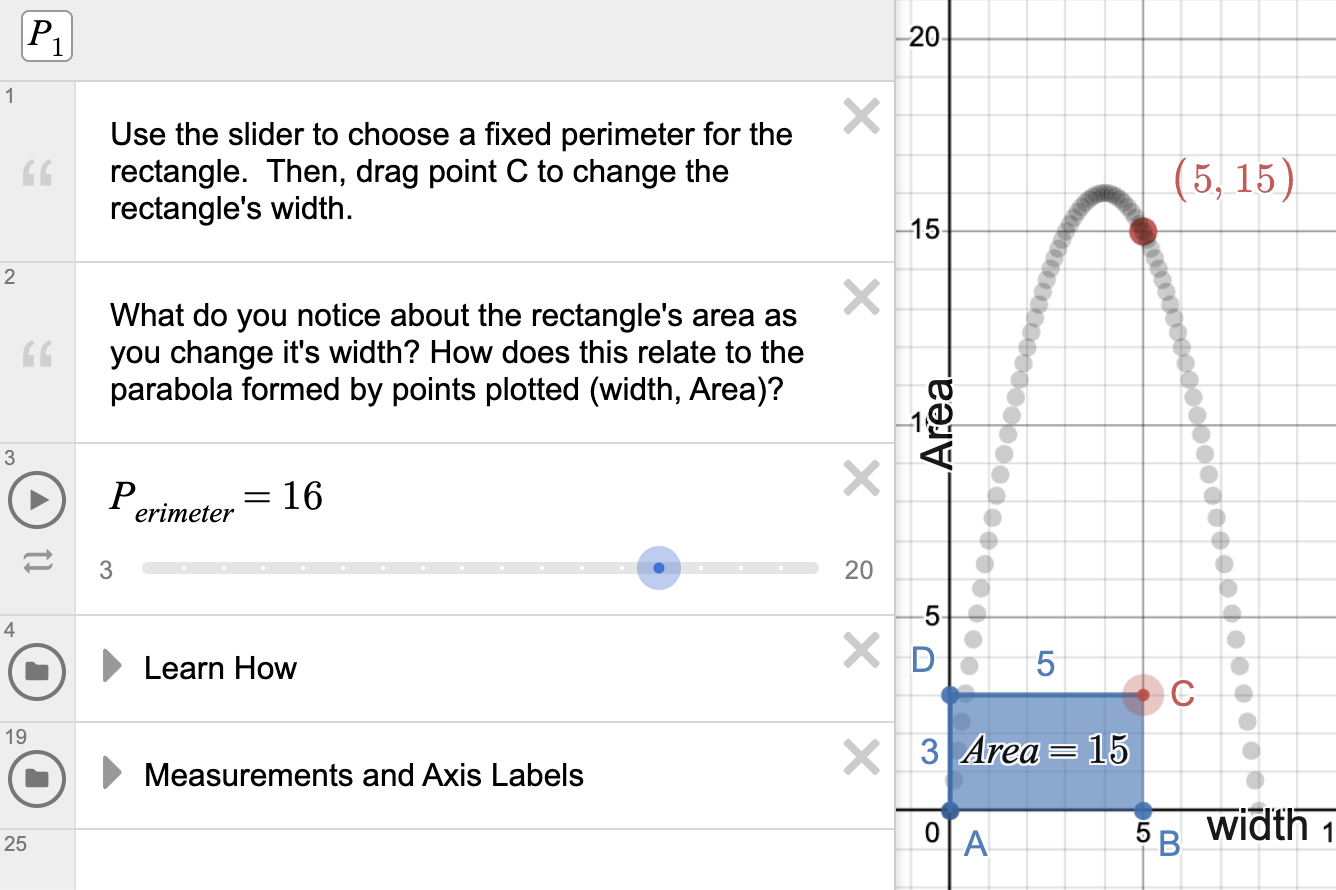# Connections Between Geometry and Algebra

The Desmos Geometry Tool allows students to explore and move between concrete and abstract representations of geometric objects. Since the Desmos Graphing Calculator is built into the Geometry Tool, students can measure, compute, work with compound transformations, and more. Students can construct in a blank workspace, on a grid, in the coordinate plane, or even with an image as a background. By using sliders, lists, and algebraic functions in their constructions, they can notice, wonder, make generalizations, and build deeper understanding of geometric and algebraic relationships.

Help your students make these connections by exploring some of the fun algebraic applications in the examples below.

### Make Dynamic Transformations

##### Getting Started

In this example, you’ll see three defined rotations (one with a constant value used for the angle of rotation, and two others where a list and a slider variable are used to define the angle of rotation). Explore this graph.• Why do you think a 60 degree rotation was used to define the first rotation in this example?
• How are the slider bounds and the list values in the example similar? How are they different?

##### Dive Deeper

In Desmos Geometry, transformations are defined as functions. If you use the transformation tools from the toolbar, these functions are defined automatically in the background. When you drag a transformation token from the navigator down to the expression list, you can apply the function to other objects like you can from the tool. Or, you can use the functions to build composite transformations. Use the composite transformation graph to explore the pattern created by a rotation + dilation composition.• What would the image of the blue polygon look like if only the dilation was applied to it? What if only the rotation was applied?
• What other composite transformations can you build?

### Use Sliders to Explore Area and Perimeter

##### Getting Started

Use the triangle perimeter and area graph to explore moving object tokens to the expression list to see how the object functions were defined and to also make generalizations about relationships between edge length, angles, area, and perimeter of a triangle.• Which location of the glider point leads the largest perimeter of the triangle? How about the smallest perimeter?
• What do you notice about the area of the triangle as the slider plays?

##### Dive Deeper

The expression list can also be used to help students see geometric relationships algebraically. Open the Maximize the Area of a Rectangle graph and use the slider to choose a fixed perimeter for the rectangle. Then, drag point C to change the rectangle's width. Use this graph to explore maximum area of a rectangle given a fixed perimeter. This graph can also be used to learn more about using the ‘with’ substitution function in Desmos.